Lagrange multiplier-y learning time

After the immensely-practical torque calculation series, let’s do something completely different: intricacies of numerical analysis. Yay!

Are you familiar with Lagrange multipliers? Besides their obvious use in optimization, they are also quite handy in finite element analysis. Like in optimization, their purpose in FEA is enforcing boundary conditions and other constraints. However, to get correct results, it’s kinda important that you don’t just blindly throw them into the mix.

### Sliding interface

I first came across them in an electric motor simulation. They were used to enforce continuity between separate stator and rotor meshes, across a so-called sliding interface. The continuity was defined based on something called the polynomial interpolation method, but that’s not important right now. What is important is that the continuity condition looked like something as simple as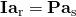.

In other words, the potential on rotor-side of airgap was set to equal to stator-side.

In addition, the basic FEA equation of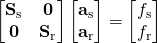of course had to be satisfied.

Now, I was in a hurry to get things done, so I dug only a little deeper. I found out that I could simply introduce new variables (let’s call them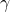), and solve the following system instead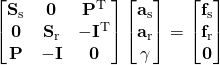.

That worked, so I mentally filed it under ‘Matrix Magic’ and never thought about it until much, much later.

By the way, that’s exactly the very same sliding interface condition that I mentioned in the numerical torque computation post.

##### 3D analysis

That time came when I was considering some 3D analysis, using the vector potential (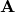) formulation. To get a unique solution, the following gauge condition should be satisfied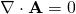.

I happened to know that an easy way to achieve this would be with Lagrange multipliers, again. So, it’d make sense that the final matrix equation would look like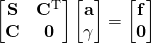.

In other words, simply add the constraint equationin matrix form, and then some matrix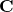to couple them together. This is how it would work, right?

Right?

Except it wouldn’t.

Why?

Now that’s the question.

### Physical meaning of lil-L

And to understand that, we have dig deeper into the physical meaning of, well, everything. So not simply Matrix Magic anymore – yuck!

Let’s begin with the gauge condition.

It’s there because the vector potential isn’t unique in 3D. We can add any gradient of a scalar potential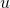to it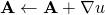and still get the same field solution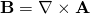.

It other words,can be thought to contain an unknown gradient field.

Now, the correct approach is to include this gradient field as an unknown – to solve bothandand then require that their sum satisfies the gauge condition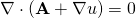.

By looking at the two equations, it’s quite easy to guess that the resulting matrix equation will look like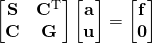.

So, we have an extra block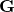in the lower right corner.

Furthermore, now we understand why everything is as it is. The Lagrance multipliers (now called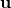!) aren’t an artificial constraint anymore. Instead, they directly define a potential, in effect cancelling out the gradient part hidden inside A.

##### Original example

So, how does this relate to out original example?

Well, it turns out the multipliershave a clear physical meaning there as well. Specifically, they add an additional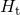– tangential component of the magnetic field on the airgap boundaries of the stator and rotor. Hence the appearance of the matrices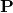and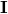on the first two rows of the linear system.

Moreover, the tangential field is defined to be such that the solution is then continuous over the airgap interface. Thus the third, extra row.

Wondering why get the relatively weird tangential field? That’s simply a peculiarity of the vector potential. For many other problems, we’d see a normal component – such as a heat flux for thermal problems, or a boundary force (Łukasz might have a word or two to say about my choice of term – sorry!) for mechanics.

##### Another case

Analysing an electric machine with circuit connections? Whether you know it or not (still using black-box commercial software?), some voltages are being solved along with the field quantities. And know what, these voltages are are in fact nothing more than Lagrange multipliers for the field problem.

### Conclusion

• Lagrange multipliers are a handy way of adding constraints to an FEA problem.
• Despite their misleading name, the multipliers do indeed have a physical meaning, too.
• Blindly adding constraints (ignoring the point above) can lead to an incorrect solution.

Need help with electric motor design or design software? Let's get in touch - satisfaction guaranteed!Lagrange multipliers and their physical meaning

### 5 thoughts on “Lagrange multipliers and their physical meaning”

•Ravi says:

Hi,

Interesting post. I am curious is it the same way periodic boundary conditions can be enforced.
For eg. In machines antiperiodic boundary conditions are let say a1=p* a2 . Here for anti periodicity p=-1. (provided node positions are similar). However in case of a random mesh at boundaries it can p will be some matrix. Will it be used as langrange multiplier?

1.Antti (admin) says:

Hi Ravi, periodicity conditions can indeed also be enforced with Lagrange multipliers.

And yes, P will indeed be a matrix if the nodes on the two boundaries won’t match 1-on-1. Or, to be precise, it will be a matrix in any case – but with matching nodes it will simply be a permuted identity matrix with zero columns. It’s probably easiest to understand with Matlab notation:

Let n_periodic_1 and n_periodic_2 be the indices of the nodes on the two periodic boundaries (ordered so that they match 1-on-1), and n = numel(n_periodic_1), and N = number of total nodes. Then, following the notation in the Sliding interface paragrap, the matrices are as follows:

I = sparse(1:n, n_periodic_1, ones(1, n), n, N)
P = sparse(1:n, n_periodic_2, periodicity_coeff*ones(1,n), n, N)

Now, in case the nodes don’t match, I will still be the same, but P will be a more complex interpolation or mortar matrix.

Hope that clarified something 😀

2.Kirill says:

Ravi, you can have a look at this paper:
M. Aubertin et al. “Periodic and Anti-Periodic Boundary Conditions With the Lagrange Multipliers in the FEM”, IEEE Trans. Magn., v. 46, no. 8

They describe the use of Lagrange multiplier approach to periodic boundary conditions quite detailed.

1.Antti (admin) says:

Thanks on my behalf! 🙂

•ravi sundaria says:

Thanks for the description